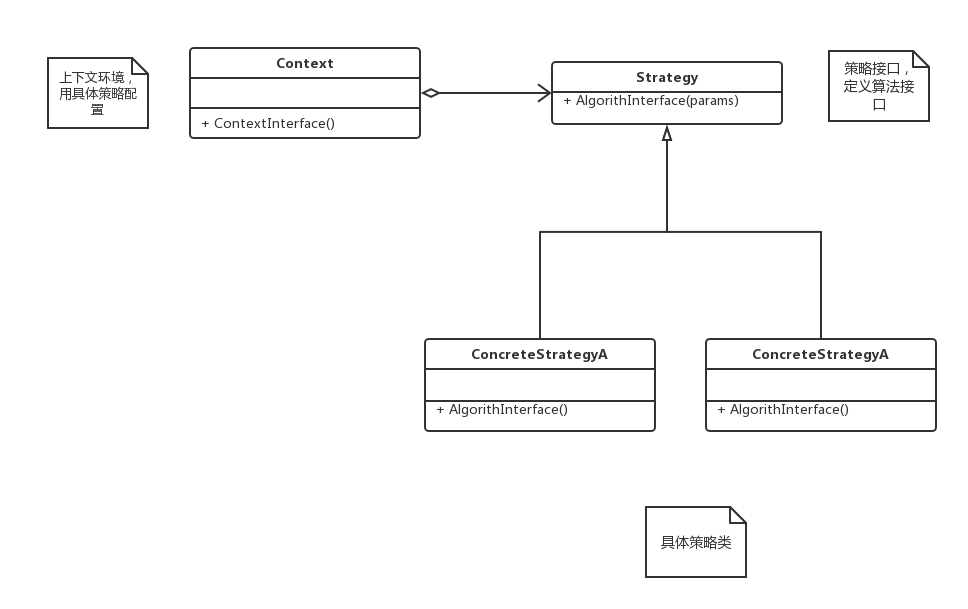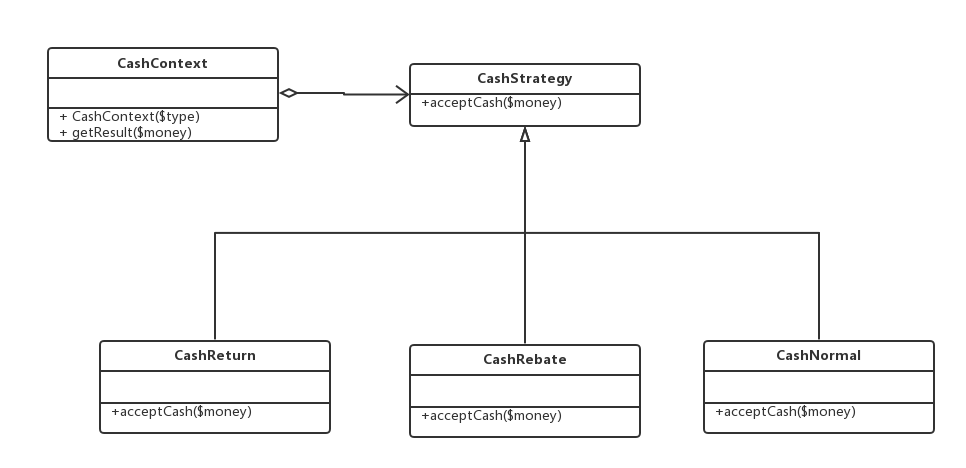# PHP常用设计模式之策略模式

#### 策略模式的特点

##### 策略模式的初衷
• 完成一项任务，往往有多种不同的方式，每一种方式我们可以称之为一种策略，我们可以根据上下文环境或者条件的不同选择不同的策略，来完成这个任务（在开发中我们会遇到这种情况，实现某一个功能，有多种途径此时可以使用策略模式）。
• 在实现某一功能时，有多种解决方法，但每一种方式执行的条件不同，我可以通过条件判断将每一种方式以硬编码的方式写入，但当某一个条件下的解决方法需要改变（新增）时，则需要改动代码（添加代码），这破坏了开放封闭原则。
##### 策略模式使用场景
• 如果在一个系统中有许多类，他们之间的区别仅在与它们的行为，那么使用策略模式可以动态地让一个对象在许多行为中选择一种行为。
• 一个系统需要动态地在几种算法中选择一种。
• 不希望客户端知道复杂的、与算法相关的数据结构，在具体策略类中封装算法和相关的数据结构，提高算法的保密性与安全性。
##### 策略模式 UML 图##### 代码

Strategy 类（抽象类也可以是接口）定义公共算法接口:

<?php
namespace DesignPattern\Strategy;

abstract class Strategy {
public abstract function algorithmInterface();
}

ConcreteStrategy 类封装具体算法逻辑:

<?php
namespace DesignPattern\Strategy;

class ConcreteStrategyA extends Strategy {

public function algorithmInterface() {
// TODO: Implement algorithmInterface() method.
}
}

ContextConcreteStrategy 来配置维护 Strategy 对象:

<?php
namespace DesignPattern\Strategy;

class Context {
/***
* @var Strategy
*/
private $strategy; /*** * @param Strategy$strategy
*/
public function Context(Strategy $strategy) {$this->strategy = $strategy; } public function ContextInterface() {$this->strategy->algorithmInterface();
}
}

#### 示例代码

- 打折
- 正常收费
- 返利收费

##### UML 图##### 代码实现

CashStrategy：定义统一的算法接口

<?php

namespace DesignPattern\Strategy;

abstract class CashStrategy {
public abstract function acceptCash($money); } CashReturn：返现算法 <?php namespace DesignPattern\Strategy; class CashReturn extends CashStrategy { private$return_money;
private $price_standard; public function __construct($price_standard, $return_money) {$this->return_money = $return_money;$this->price_standard = $price_standard; } public function acceptCash($money) {
if ($money >=$this->price_standard) {
return $money -$this->return_money;
}
return $money; } } CashNormal：正常情况算法 <?php namespace DesignPattern\Strategy; class CashNormal extends CashStrategy { public function acceptCash($money) {
return $money; } } CashRebate：打折算法 <?php namespace DesignPattern\Strategy; class CashRebate extends CashStrategy { private$discount;

public function __construct($discount) {$this->discount = $discount; } public function acceptCash($money) {
return $money *$this->discount;
}
}

CashContext：上下文环境类，根据算法类型生成不同的策略类。

<?php

namespace DesignPattern\Strategy;

class CashContext {

const CASH_TYPE_NORMAL = 1;
const CASH_TYPE_RETURN = 2;
const CASH_TYPE_REBATE = 3;

/***
* @var CashStrategy
*/
private $cash_strategy; public function CashContext($type) {
switch ($type) { case self::CASH_TYPE_NORMAL:$this->cash_strategy = new CashNormal();
break;
case self::CASH_TYPE_RETURN:
$this->cash_strategy = new CashReturn(200, 200); break; case self::CASH_TYPE_REBATE:$this->cash_strategy = new CashRebate(0.8);
break;
}
}

public function getResult($money) { return$this->cash_strategy->acceptCash($money); } } 注意 这里将策略模式简单工厂模式结合，这么做的好处是很明显的，从使用者的角度来说，只需要知道有哪些算法类型即可，不需要根据具体实例化某种策略；从设计的角度来说，这实现了代码间的解耦，减少了使用者代码和算法代码的关联程度 StrategyTest：测试 <?php namespace DesignPattern\Strategy\Tests; use DesignPattern\Strategy\CashContext; use PHPUnit\Framework\TestCase; class StrategyTest extends TestCase { public function testStrategy() { try {$cash_context = new CashContext();
$cash_context->CashContext(CashContext::CASH_TYPE_NORMAL);$this->assertEquals(500, $cash_context->getResult(500));$cash_context->CashContext(CashContext::CASH_TYPE_REBATE);
$this->assertEquals(400,$cash_context->getResult(500));

$cash_context->CashContext(CashContext::CASH_TYPE_RETURN);$this->assertEquals(300, $cash_context->getResult(500)); } catch (\Exception$e) {
echo \$e->getMessage();
}
}
}

#### 总结

• 策略模式是对算法的封装，它把算法的责任和算法本身分割开，委派给不同的对象管理。策略模式通常把一个系列的算法封装到一系列的策略类里面，作为一个抽象策略类的子类。
• 策略模式主要优点在于对「 开闭原则 」的完美支持，在不修改原有系统的基础上可以更换算法或者增加新的算法，它很好地管理算法族，提高了代码的复用性，是一种替换继承，避免多重条件转移语句的实现方式；其缺点在于客户端必须知道所有的策略类，并理解其区别，同时在一定程度上增加了系统中类的个数，可能会存在很多策略类。